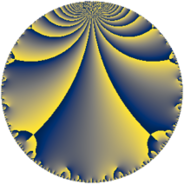# Properties

 Label 225.6.mLevel $225$ Weight $6$ Character orbit 225.m Rep. character $\chi_{225}(19,\cdot)$ Character field $\Q(\zeta_{10})$ Dimension $248$ Sturm bound $180$

# Learn more about

## Defining parameters

 Level: $$N$$ $$=$$ $$225 = 3^{2} \cdot 5^{2}$$ Weight: $$k$$ $$=$$ $$6$$ Character orbit: $$[\chi]$$ $$=$$ 225.m (of order $$10$$ and degree $$4$$) Character conductor: $$\operatorname{cond}(\chi)$$ $$=$$ $$25$$ Character field: $$\Q(\zeta_{10})$$ Sturm bound: $$180$$

## Dimensions

The following table gives the dimensions of various subspaces of $$M_{6}(225, [\chi])$$.

Total New Old
Modular forms 616 256 360
Cusp forms 584 248 336
Eisenstein series 32 8 24

## Trace form

 $$248q + 5q^{2} + 989q^{4} + 180q^{5} + 620q^{8} + O(q^{10})$$ $$248q + 5q^{2} + 989q^{4} + 180q^{5} + 620q^{8} + 355q^{10} + 250q^{11} - 5q^{13} - 2251q^{14} - 12111q^{16} - 950q^{17} - 1193q^{19} + 10065q^{20} + 3990q^{22} - 10985q^{23} + 260q^{25} - 10638q^{26} - 4005q^{28} + 7109q^{29} - 11301q^{31} + 6277q^{34} - 6105q^{35} - 8250q^{37} + 8655q^{38} - 76370q^{40} + 388q^{41} - 15934q^{44} - 2503q^{46} - 58740q^{47} - 549958q^{49} - 98505q^{50} + 64640q^{52} + 45850q^{53} - 31510q^{55} - 72820q^{56} - 45550q^{58} + 49788q^{59} - 41661q^{61} + 3090q^{62} + 31804q^{64} + 37645q^{65} - 184540q^{67} - 5730q^{70} - 108978q^{71} + 18975q^{73} + 82704q^{74} + 65416q^{76} + 13430q^{77} + 28191q^{79} - 296510q^{80} - 309165q^{83} - 393645q^{85} + 221265q^{86} - 1357130q^{88} + 57607q^{89} - 182478q^{91} + 813060q^{92} + 192285q^{94} - 243015q^{95} + 681330q^{97} - 529400q^{98} + O(q^{100})$$

## Decomposition of $$S_{6}^{\mathrm{new}}(225, [\chi])$$ into newform subspaces

The newforms in this space have not yet been added to the LMFDB.

## Decomposition of $$S_{6}^{\mathrm{old}}(225, [\chi])$$ into lower level spaces

$$S_{6}^{\mathrm{old}}(225, [\chi]) \cong$$ $$S_{6}^{\mathrm{new}}(25, [\chi])$$$$^{\oplus 3}$$$$\oplus$$$$S_{6}^{\mathrm{new}}(75, [\chi])$$$$^{\oplus 2}$$